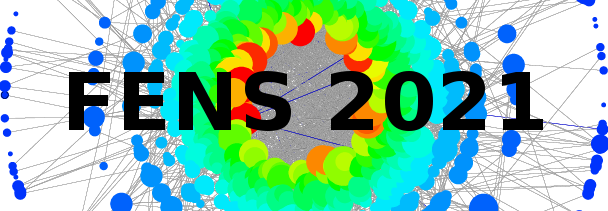#11th Polish Symposium on Physics in Economy and Social Sciences

1-3 July 2021
ONLINE
Europe/Warsaw timezone

## Discontinous phase transitions in the generalized q-voter model on random graphs

2 Jul 2021, 17:07
1m
ONLINE

Poster

### Speakers

Jakub Pawłowski (Wrocław University of Science and Technology) Mr Arkadiusz Lipiecki (Wrocław University of Science and Technology)

### Description

We investigate the binary $q$-voter model with generalized anticonformity on random Erdős–Rényi graphs. The generalization refers to the freedom of choosing the size of the influence group independently for the case of conformity $q_c$ and anticonformity $q_a$. This model was studied before on the complete graph, which corresponds to the mean-field approach, and on such a graph discontinuous phase transitions were observed for $q_c>q_a + \Delta q$, where $\Delta q=4$ for $q_a \le 3$ and $\Delta q=3$ for $q_a>3$. Examining the model on random graphs allows us to answer the question whether a discontinuous phase transition can survive the shift to a network with the value of average node degree that is observed in real social systems. By approaching the model both within Monte Carlo (MC) simulations and Pair Approximation (PA), we are able to compare the results obtained within both methods and to investigate the validity of PA. We show that as long as the average node degree of a graph is relatively large, PA overlaps MC results. On the other hand, for smaller values of the average node degree, PA gives qualitatively different results than Monte Carlo simulations for some values of $q_c$ and $q_a$. In such cases, the phase transition observed in the simulation is continuous on random graphs as well as on the complete graph, whereas PA indicates a discontinuous one. We determine the range of model parameters for which PA gives incorrect results and we present our attempt at validating the assumptions made within the PA method in order to understand why PA fails, even on the random graph.

### Primary authors

Jakub Pawłowski (Wrocław University of Science and Technology) Mr Arkadiusz Lipiecki (Wrocław University of Science and Technology)

### Co-authors

Katarzyna Sznajd-Weron (Department of Theoretical Physics, Faculty of Fundamental Problems of Technology, Wroclaw University of Science and Technology) Mrs Angelika Abramiuk-Szurlej (Wrocław University of Science and Technology)

### Presentation Materials

 29_Pawlowski.pdf Meeting ID: 307 287 8552 / Passcode: 359927